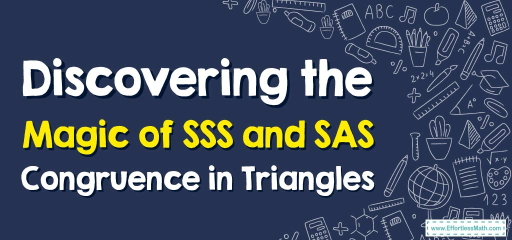# Discovering the Magic of SSS and SAS Congruence in Triangles

Triangles are one of the most fundamental shapes in geometry. But what happens when we start comparing them? Can we say that two triangles are essentially "the same" based on their sides and angles? This is where the concepts of SSS (Side-Side-Side) and SAS (Side-Angle-Side) congruence come into play. Let's delve deeper into these interesting postulates! Congruence in geometry refers to two objects being identical in shape and size. In the realm of triangles, we often look at the lengths of their sides and the magnitudes of their angles to determine if they are congruent. Specifically, the SSS and SAS postulates provide shortcuts to confirm congruence without examining every single aspect of two triangles.## Step-by-step Guide: SSS and SAS Congruence

1. SSS (Side-Side-Side) Congruence Postulate:
If three sides of one triangle are congruent to three sides of another triangle, then the two triangles are congruent. To use this postulate:

• Ensure you know the lengths of all three sides of both triangles.
• Compare each corresponding side.
• If all three sides in one triangle are equal in length to the three sides of the other triangle, the two triangles are congruent.

2. SAS (Side-Angle-Side) Congruence Postulate:
If two sides and the included angle of one triangle are congruent to two sides and the included angle of another triangle, then the triangles are congruent. To use this postulate:

• Ensure you know the lengths of two sides and the magnitude of the included angle for both triangles.
• Compare the two sides and the included angle.
• If both conditions are met, then the two triangles are congruent.

### Examples

Example 1:
Are triangles with sides $$4 \text{ cm}$$, $$5 \text{ cm}$$, and $$6 \text{ cm}$$ and $$4 \text{ cm}$$, $$5 \text{ cm}$$, and $$6 \text{ cm}$$ congruent based on the SSS postulate?

Solution:
Both triangles have sides of:
$$4 \text{ cm}, 5 \text{ cm}, \text{ and } 6 \text{ cm}$$
Since the sides match exactly, the two triangles are congruent by the SSS postulate.

Example 2:
Are triangles with sides $$7 \text{ cm}$$ and $$8 \text{ cm}$$, and an included angle of $$60^{\circ}$$, and another triangle with sides $$7 \text{ cm}$$ and $$8 \text{ cm}$$, with an included angle of $$60^{\circ}$$ congruent based on the SAS postulate?

Solution:
Both triangles have:
Sides of $$7 \text{ cm} \text{ and } 8 \text{ cm}$$
An included angle of $$60^{\circ}$$
Since both the sides and the included angles match, the two triangles are congruent by the SAS postulate.

### Practice Questions:

1. Are triangles with sides $$10 \text{ cm}$$, $$12 \text{ cm}$$, and $$15 \text{ cm}$$ and $$10 \text{ cm}$$, $$12 \text{ cm}$$, and $$14 \text{ cm}$$ congruent by the SSS postulate?
2. Are triangles with sides $$9 \text{ cm}$$ and $$11 \text{ cm}$$, and an included angle of $$45^{\circ}$$, and another triangle with sides $$9 \text{ cm}$$ and $$11 \text{ cm}$$, with an included angle of $$45^{\circ}$$ congruent by the SAS postulate?

1. No
2. Yes

### What people say about "Discovering the Magic of SSS and SAS Congruence in Triangles - Effortless Math: We Help Students Learn to LOVE Mathematics"?

No one replied yet.

X
30% OFF

Limited time only!

Save Over 30%

SAVE $5 It was$16.99 now it is \$11.99